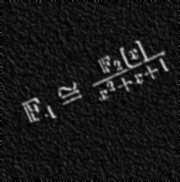# Lomont.org

(Also lomonster.com and clomont.com)

Published

Summary

# Finite FieldsBelow are tables of all irreducible polynomials I computed with Mathematica 4.0. Each list is the following format:

(the example is the characteristic 2 fields, degree n = 3 polys)

Attributes[result$76499] = {Temporary} result$76499 = {3, 2, 2, 2, 0.016000000000019554*Second, {"101", "011"}}

the first 5 numbers are the degree, characteristic, number of expected irreducible polynomials, the number actually found (which should agree), and the time it took my PIII 500 to do the computation.

The "101" and "011" are the irreducible polynomial coefficients, and the leading terms of each poly is dropped (since monic) to save space, so these represent the polynomials x^3 + x^2 + 1 and x^3 + x + 1

letters A-Z in the coefficients are for numbers 10 to 35 for primes  up to 31

n below denotes the degree of the polynomials

A zip file (~900K) of all fields is available.

More fields will be added since I need them for some conjectures. They are just slow to compute.

Please email me if you find any errors.

 Characteristic 2 fields n = 1 n = 2 n = 3 n = 4 n = 5 n = 6 n = 7 n = 8 n = 9 n = 10 n = 11 n = 12 n = 13 n = 14 n = 15 n = 16 n = 17 n = 18 n = 19 n = 20 Characteristic 3 fields n = 1 n = 2 n = 3 n = 4 n = 5 n = 6 n = 7 n = 8 n = 9 n = 10 Characteristic 5 fields n = 1 n = 2 n = 3 n = 4 n = 5 n = 6 n = 7 n = 8 n = 9 n = 10 Characteristic 7 fields n = 1 n = 2 n = 3 n = 4 n = 5 n = 6 n = 7 n = 8 n = 9 n = 10 Characteristic 11 fields n = 1 n = 2 n = 3 n = 4 n = 5 n = 6 n = 7 n = 8 n = 9 n = 10 Characteristic 13 fields n = 1 n = 2 n = 3 n = 4 n = 5 n = 6 n = 7 n = 8 n = 9 n = 10 Characteristic 17 fields n = 1 n = 2 n = 3 n = 4 n = 5 n = 6 n = 7 n = 8 n = 9 n = 10 Characteristic 19 fields n = 1 n = 2 n = 3 n = 4 n = 5 n = 6 n = 7 n = 8 n = 9 n = 10 Characteristic 23 fields n = 1 n = 2 n = 3 n = 4 n = 5 n = 6 n = 7 n = 8 n = 9 n = 10 Characteristic 29 fields n = 1 n = 2 n = 3 n = 4 n = 5 n = 6 n = 7 n = 8 n = 9 n = 10 Characteristic 31 fields n = 1 n = 2 n = 3 n = 4 n = 5 n = 6 n = 7 n = 8 n = 9 n = 10

The End - Main Page

Categories:

Tags: